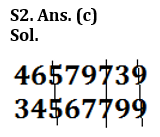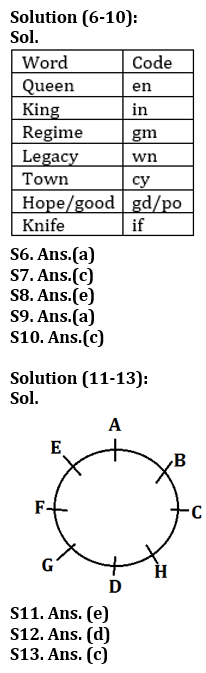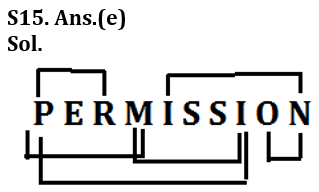Latest Banking jobs   »

# Reasoning Ability Quiz For IDBI AM/ Bank of India PO 2023 -11th March

Q1. What should come in place of question mark (?) in the following series based on the above arrangement?
ZA YB XC WD ?
(a) EU
(b) LO
(c) LP
(d) VE
(e) None of these

Q2. How many numbers will remain at the same position in the given number “46579739” when they are arranged in the ascending order from left to right?
(a) One
(b) Two
(c) Three
(d) More than three
(e) None of these.

Q3. If it is possible to make only one meaningful word with the 1st ,2nd ,4th and 7th letters of the word ‘CAREERPOWER’ which would be the third letter of the word from the right? If more than one such word can be formed give ‘Y’ as the answer. If no such word can be formed, give ‘Z’ as your answer.
(a) Y
(b) P
(c) C
(d) Z
(e) M

Q4. If 1 is added to each odd digit and 2 is subtracted from each even digit in the number 1436587, then how many digits will appear twice in the new number thus formed?
(a) Only 8
(b) Only 8 and 6
(c) 8, 6 and 4
(d) 2, 4 and 6
(e) None of these

Q5. How many letters will be remaining at the same position in the word ‘NIGHTKING’ when letters arranged according to alphabetical order from left to right?
(a) One
(b) Two
(c) Three
(d) More than Three
(e) None

Directions (6-10): Study the following information carefully and answer the questions given below:
In a certain code language:
‘Queen King Regime’ is written as ‘en in gm’,
‘Regime legacy town’ is written as ‘gm cy wn’,
‘Hope town good’ is written as ‘cy gd po’
‘Regime King Knife’ is written as ‘in gm if’.

Q6. What is the code for ‘legacy knife’ in the given code language?
(a) wn if
(b) gm wn
(c) gd wn
(d) if cy
(e) None of these

Q7. What is the code for ‘Regime’ in the given code language?
(a) en
(b) in
(c) gm
(d) if
(e) None of these

Q8. What is the code for ‘Good’ in the given code language?
(a) gd
(b) po
(c) cy
(d) if
(e) Can’t be determined

Q9. What may be the code for ‘King Hope’ in the given code language?
(a) in gd
(b) if po
(c) in cy
(d) Either (a) or (c)
(e) None of these

Q10. What is the code for ‘Town’?
(a) gd
(b) po
(c) cy
(d) in
(e) None of these

Directions (11-13): Study the information carefully and answer the questions given below.
Eight persons A, B, C, D, E, F, G, and H are sitting in a circle facing center. B sits third to right of D. E is not immediate neighbor of D. E faces H. C sits third to the left of E. Only one person sits between A and F who faces C.

Q11. Which of the following sits second to the right of G?
(a) C
(b) D
(c) B
(d) A
(e) None of these

Q12. How many persons sit between C and E when counted from the left of C?
(a) Two
(b) One
(c) Three
(d) Four
(e) None of these

Q13. Which of the following pair is an immediate neighbor of A?
(a) F, C
(b) B, D
(c) E, B
(d) H, C
(e) Can’t be determined

Q14. Aman is 21st from the left end of a row of 47 students and Monika is 23rd from the right end in the same row. How many students are there between them in the row?
(a) 1
(b) 3
(c) 2
(d) 5
(e) None of these

Q15. How many pairs of letters are there in the word “PERMISSION” each of which have as many letters between them in the word as they have between them in the English alphabetical series?
(a) Two
(b) Five
(c) Three
(d) Four
(e) More than five

Solutions

S1. Ans. (d)
Sol. VES3. Ans. (a)
Sol. Pace, Cape

S4. Ans. (d)
Sol. 1436587
2244668

S5. Ans.(b)
Sol. NIGHTKING
GGHIIKNNTS14. Ans. (b)
Sol. Aman position from right end = (47+1-21) = 27
Students between them = (27-23-1) = 3## FAQs

### What is the selection process of the IDBI AM?

The selection process of IDBI AM is Online exam and Interview.

#### Congratulations!Union Budget 2023-24: Free PDF